We've updated our prices on products from many online Montessori stores! If we've missed your favorite store, please let us know!
Math - Introduction to the Decimal System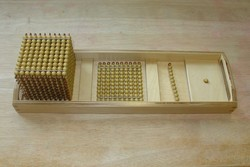LevelPrimary
Age4+
PrerequisitesCards and Counters
1 ten bar
1 hundred square
1 thousand cube
tray

This activity is the child's introduction to the Golden Bead material. This material is a concrete representation of the decimal system.

## Presentation

1. Take the tray to a table or mat. Be sure that the thousand cube is on the left and the unit bead is on the right.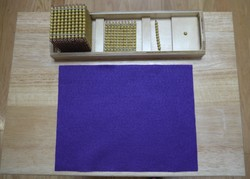2. Take out the unit bead. Place it to the right of the mat. "This is one. We also call it a unit."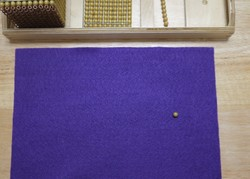3. Take out the ten bar. Place it vertically to the left of the unit. "This is ten."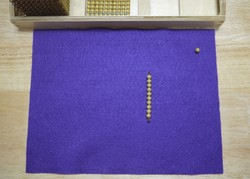4. Take the unit bead and count over the units in the ten bar. "Let's see how many unit beads there are in a ten. One, two, three... There are ten units in a ten.”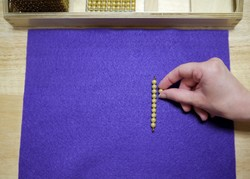5. Take out the hundred square, place it to the left of the ten bar, with the wires vertical. "This is a hundred."6. Using the ten bar, count over the tens in the hundred square. "Let's see how many tens there are in a hundred. One ten, two tens, three tens... There are ten tens in a hundred."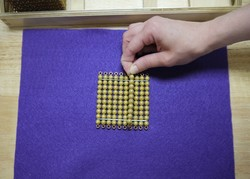7. Take out the thousand cube, place it to the left of the hundred square with the wires vertical to the mat. "This is a thousand."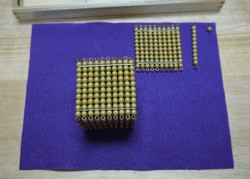8. Take the hundred square and count the hundreds in the thousand cube. Go from bottom to top. "Let's see how many hundreds there are in a thousand. One hundred, two hundreds, three hundreds... There are ten hundreds in a thousand."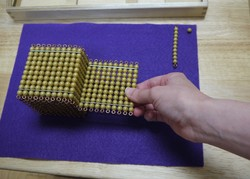9. Lay out all of the beads and name them one more time.
10. Do a three period lesson.

## Points of Interest

• The large place values can be exciting to children.
• Seeing how heavy the thousand is.

## Control of Error

• The directress' and the child’s own knowledge

## Variations and Extensions

At the elementary level you can introduce the decimal cards at the same time.

## Material

- A tray containing 1 golden bead unit, 1 golden ten-bar, 1 golden hundred square and 1 thousand cube. - A small mat for the table.

Prices and links checked: 23 January 2022
(Amazon/Bookshop are affiliate links, which help cover costs of running the site.)

Store Price
Affordable Montessori \$49.00
Alison's \$93.71
Bruins \$134.00
E & O \$73.80
IFIT \$24.00
Materials Co. of Boston \$78.00
Montessori Concepts \$53.00
Montessori n Such \$75.00
Montessori Outlet \$54.95
Nienhuis